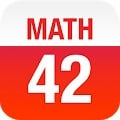# MATH 42 APK

MATH 42 helps over 2.200.000 middle-school, high-school and college students, to solve their math problems and get better grades at the fraction of the cost of a private tutor. MATH 42 helps with (1) intelligent approaches to the solution, (2) step-by-step solutions of their problems (3) an Assessment Center.

MATH 42 is a trusted mathematical resource around the world and the numbers prove it: Over 2.2 million downloads, used on over 450.000 tablets in schools, in cooperation with the biggest educational publisher in Germany (Klett).

FEATURES

• Intuitive entry of formulas
• Intelligent suggestions on how to approach a problem (unique worldwide)
• Detailed step-by-step solutions, that adapt to a student’s need (unique worldwide)
• Extensive corresponding mathematical explanations with examples
• Interactive graphs, that visualize problems
• Automatically generated and curated assessment, that enables and facilitates rapid progress
• Instant-Calculator, that computes during the entry

ASSESSMENT CENTER

• Exercises — subdivided by topic and level
• Training mode — afterwards, each problem can be analyzed step-by-step
• Test mode — enabling the identification of strengths and weaknesses
• Test results and statistics show progress over time
• Tests and exercises were created in cooperation with experienced pedagogues and private tutors

MATHEMATICAL FEATURES

Term Transformation
• Simplyfing and expanding
• Factorization (Factoring out, application of the binomial formulas)
• Transforming powers and roots
• Adding fractions, multiplying fractions, reducing fractions, expanding fractions
• Computing the gcd and the lcm
• Trigonometric transformations (Is being enhanced)
• Logarithmic transformations (Is being enhanced)

Functions
• Roots
• Y-axis intersection
• Domain of definition
• Extremes (Maximums, Minimums)
• Inflexion points
• Curve sketching
• Derivation
• Fundamental derivatives,
• Factor rule
• Sum rule
• Product rule
• Quotient rule
• Chain rule
• Integration
• Fundamental integrals,
• Integration by substitution
• Integration by parts
• Partial fraction decomposition

Equations
• Linear equations
• Special equations of higher degree
• Linear systems of equations
• Non-linear system of equations

Matrices
• Multiplying matrices
• Transposing matrices
• Inverting matrices
• Determinants
• Characteristic polynomials
• Eigenvalues
• Eigenspaces
• Kernels
• Ranks
• Cross-product
• Gaussian elimination

TECHNOLOGY

• MATH 42 works without templates or pre-calculated solutions
• MATH 42 solves all problems on the fly, like a teacher at the board
• MATH 42 is a real symbolic algebra system
• MATH 42 bases on artificial intelligence
• MATH 42 is a completely self-developed system

#### What’s New

MATH 42 — your digital private math tutor — is completely free now!